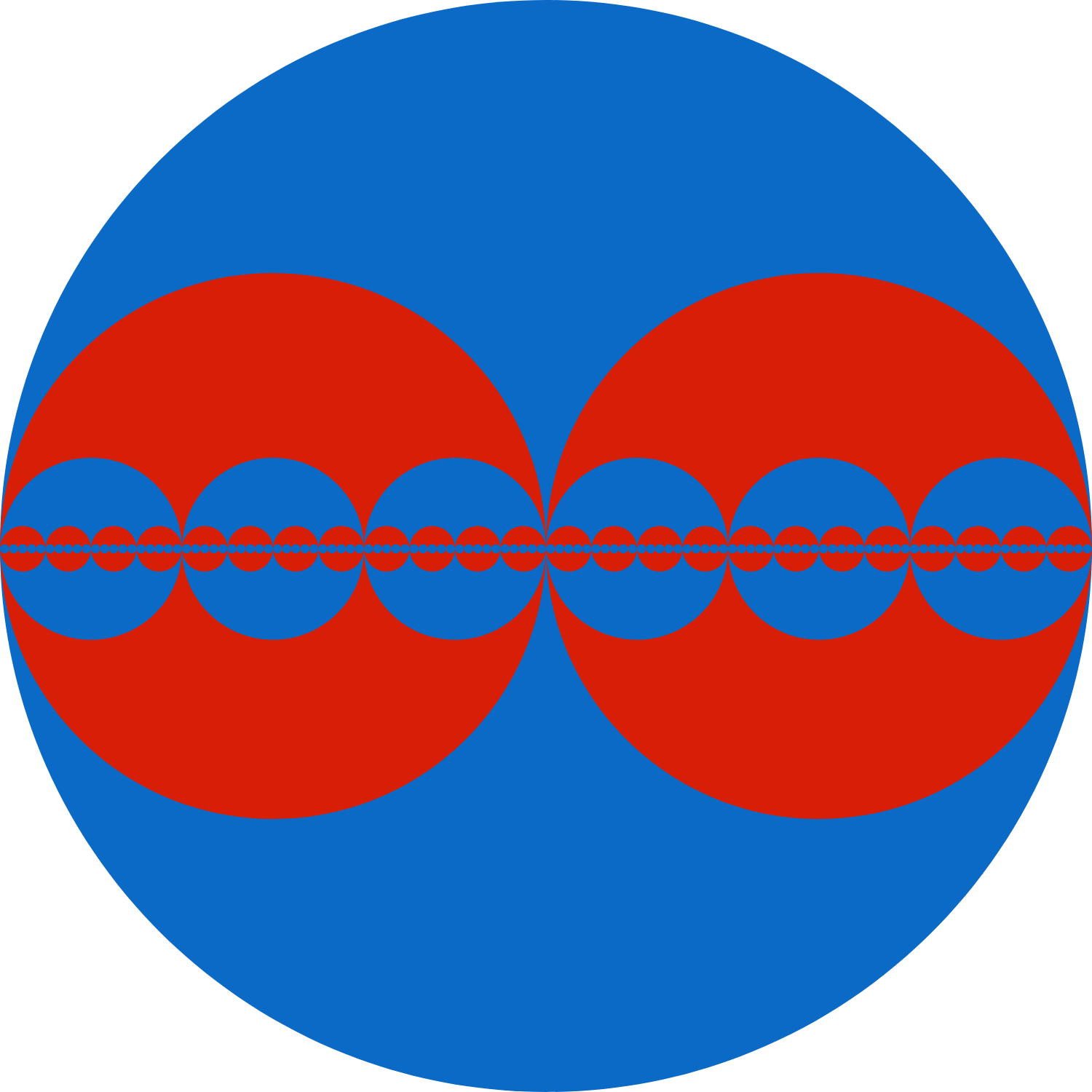# Nested Circles

Calculus Level 3In the figure above, we have a large blue circle of radius 1.
In this circle, we draw 2 equal red circles that are tangent to each other and to the large circle.
In each of these smaller red circles, we draw 3 equal blue circles in the same fashion.
In each of these smaller blue circles, we draw 4 equal red circles, and so on.

Compute the area of the red region.

×